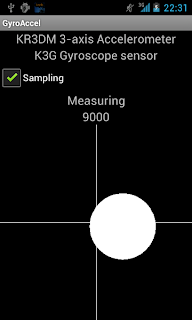# My life with Android :-)

## Friday, February 10, 2012

### Example application for accelerometer/gyroscope processing on Android

In the previous parts we have seen how to simulate the gravity vector with the gyroscope if the accelerometer is not able to measure gravity correctly (because it is subject to motion acceleration too beside the gravity acceleration) and how to update this simulated gravity vector from the accelerometer to prevent the accumulation of measurement errors. At the end of the previous post we had a gravity vector which was normally taken from the accelerometer but our algorithm switched to gyroscope simulation automatically when needed.

We need just one more step to enable cool applications and that step is very evident. If you remember my Droidcon 2011 presentation (slide 24), the acceleration measured by the accelerometer is the sum of gravity and motion acceleration. If both are present, they cannot be separated in the general case without additional sensor input. But now we have the gravity acceleration which is reasonably exact even if the accelerometer is subject to motion acceleration too. This means that we can extract the motion acceleration by subtracting the simulated gravity acceleration from the acceleration measured by the accelerometer.

This opens the way for applications based on dynamic movements much like the Wii games with Motion Plus accessory (which actually do the same as the Motion Plus accessory is really just a 2+1 axis gyroscope).

The example program can be downloaded from here.

After all these simulation script, let's see something hands-on. The example program is the implementation of all these ideas we have seen so far. You need an Android phone with gyroscope to test it. The gyroscope processing algorithm is implemented in SamplingService.java. GyroAccelActivity is really doing only visualization, getting callbacks from SamplingService.

When started, the service enters the calibration state. This state is necessary to get a good fix of the initial gravity vector so the device should not be moved when calibrating. The initial gyroscope drift experienced on my Nexus S also ceases during the calibration period.

Then the service enters the measuring state and implements everything we discussed so far. It samples the accelerometer and the gyroscope paralelly, simulates the gravity vector if it finds out that the accelerometer is probably subject to motion acceleration and calls back the activity with the calculated motion acceleration vector. The acceleration is shown as movement of a large white ball (quite ugly, actually). The x and the y components of the motion acceleration move the ball horizontally and vertically while the size of the ball is calculated from the z component of the motion acceleration.

Note that the motion acceleration is expressed in the coordinate system of the device itself, so you may get some unexpected result if the device is not parallel to the floor surface. As we have the gravity vector, rotating the motion acceleration vector to the Earth's coordinate system is simple exercise (took me several days to get it right ;-)). We calculate two rotations (around z and y axes) to rotate the gravity vector into the z axis. At the same time we rotate the motion acceleration vector with the same rotations. This yields a normalized motion acceleration vector that would have been measured if the device were parallel to the surface.

If you are done with playing the ball, you will notice that analyzing these 3D acceleration vectors are not trivial. You can try setting the SamplingService.DEBUG field to true, then the application produces the usual /sdcard/capture.csv file (beware, it can be large) that you can download to your computer and visualize with this Sage script.